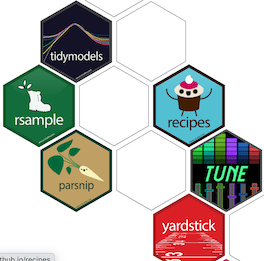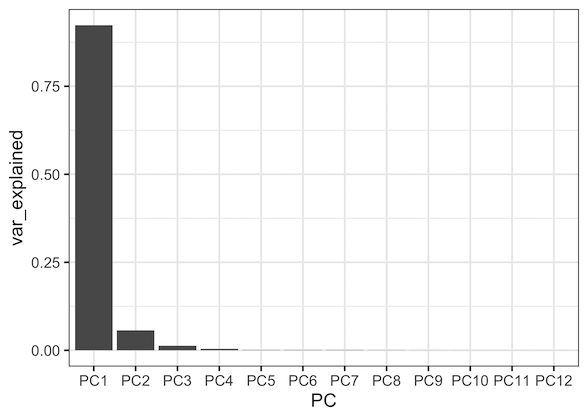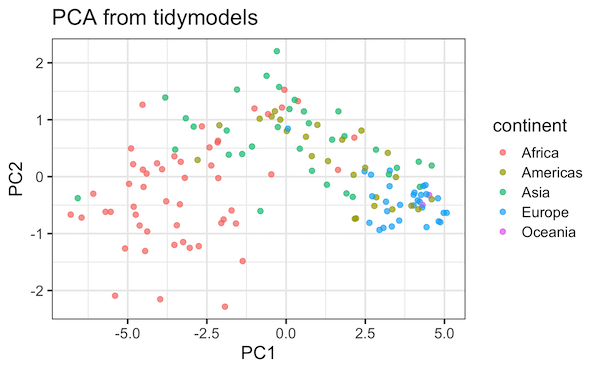# PCA with tidymodels in R

186Introduction to tidymodels with PCA

tidymodels, is one of the new suite of packages for doing machine learning analysis in R with tidy principles from RStudio.

Tidymodels, the metapackage, has a core set of packages for statistical/machine learning models like infer, parsnip, recipes, rsample, and dials in addition to the core tidyverse packages dplyr, ggplot2, purr, and broom.

In addition to providing tidy framework for common supervised learning algorithms, tidymodels also supports unsupervised learning algorithms like Principal Component Analysis (PCA).

Finally, got a chance to play with tidymodels. What better way to start tidymodels than with Principal Component Analysis (PCA). In this post we will see an example of doing PCA analysis using tidymodels. Under the hood, tidymodels uses prcomp in R for doing PCA.

```library(tidymodels)
library(tidyverse)
library(gapminder)
theme_set(theme_bw(16))

```

We will use gapminder dataset to do PCA.

```head(gapminder)
## # A tibble: 6 x 6
##   country     continent  year lifeExp      pop gdpPercap
##   <fct>       <fct>     <int>   <dbl>    <int>     <dbl>
## 1 Afghanistan Asia       1952    28.8  8425333      779.
## 2 Afghanistan Asia       1957    30.3  9240934      821.
## 3 Afghanistan Asia       1962    32.0 10267083      853.
## 4 Afghanistan Asia       1967    34.0 11537966      836.
## 5 Afghanistan Asia       1972    36.1 13079460      740.
## 6 Afghanistan Asia       1977    38.4 14880372      786.
```

We will use lifeExp values over the years from all the countries to do the PCA. To do that we will first convert the gapminder data in tidy/long form to wide form. We will use tidyr’s pivot_wider() to reshape the data.

```life_df <- gapminder %>%
select(country, continent, year, lifeExp) %>%
pivot_wider(values_from = lifeExp, names_from = year)
```

Now we have our life expectancy data in wide form with country and continent information. Note that the years are column names and the lifeExp values are rows now.

```life_df %>% head()
# # A tibble: 142 x 14
##    country continent `1952` `1957` `1962` `1967` `1972` `1977` `1982` `1987`
##    <fct>   <fct>      <dbl>  <dbl>  <dbl>  <dbl>  <dbl>  <dbl>  <dbl>  <dbl>
##  1 Afghan… Asia        28.8   30.3   32.0   34.0   36.1   38.4   39.9   40.8
##  2 Albania Europe      55.2   59.3   64.8   66.2   67.7   68.9   70.4   72
##  3 Algeria Africa      43.1   45.7   48.3   51.4   54.5   58.0   61.4   65.8
##  4 Angola  Africa      30.0   32.0   34     36.0   37.9   39.5   39.9   39.9

```

The first step in using tidymodels is to define a pipeline, i.e. a series of analysis steps, that we want to perform. R package recipies, a part of tidymodels lets you build a recipe (or a pipeline) for the analysis.

Let us start a recipe by providing the data we will be using first using recipe() function. Here we simply creating a new pipleine with reference to data.

```pca_recipe <- recipe(~., data = life_df)
```

Now we have a bare bone recipe just pointing to the data. And then we can build a recipe with series of steps that we typically do in the analysis. Our simple recipe to do PCA analysis involve three steps.

In the case of PCA, we typically center the data. We can center the data, here columns, using step_center() function. In this example, we specify to center all numeric columns.

Next, we scale the data (columns again) using step_scale() function all numeric variables in the data.

And finally, we specify that we want to do PCA using step_pca().

```pca_trans <- pca_recipe %>%
# center the data
step_center(all_numeric()) %>%
# center the data
step_scale(all_numeric()) %>%
# pca on all numeric variables
step_pca(all_numeric())
```

We have saved the recipe with the steps in a variable called pca_trans. So far we have not done any computation as such. We have just specified list of things to do. We can check the variable and learn a bit more about what we have specified.

```pca_trans
## Data Recipe
##
## Inputs:
##
##       role #variables
##  predictor         14
##
## Operations:
##
## Centering for all_numeric
## Scaling for all_numeric
## No PCA components were extracted.
```

Now we are ready for actual analysis. We will use the recipe we built so far as argument to the prep() function.

```pca_estimates <- prep(pca_trans)
```

Now tidymodels actually performs the operations that we specified before. We can see from the message below that it centered the column variables, scaled them and finally performed PCA.

```pca_estimates
## Data Recipe
##
## Inputs:
##
##       role #variables
##  predictor         14
##
## Training data contained 142 data points and no missing data.
##
## Operations:
##
## Centering for 1952, 1957, 1962, 1967, 1972, 1977, 1982, ... [trained]
## Scaling for 1952, 1957, 1962, 1967, 1972, 1977, 1982, ... [trained]
## PCA extraction with 1952, 1957, 1962, 1967, 1972, 1977, 1982, ... [trained]

```

We stored the result of the PCA analysis in the variable pca_estimates and it contains lot of information about what we have done so far.

We can check the R objects content with names() and check on each of them.

```names(pca_estimates)
##  "var_info"       "term_info"      "steps"          "template"
##  "levels"         "retained"       "tr_info"        "orig_lvls"
##  "last_term_info"
```

For example, we can check var_info and learn that it contains the information about the data we used.

```pca_estimates\$var_info

# # A tibble: 14 x 4
##    variable  type    role      source
##    <chr>     <chr>   <chr>     <chr>
##  1 country   nominal predictor original
##  2 continent nominal predictor original
##  3 1952      numeric predictor original
##  4 1957      numeric predictor original
```

Our results from PCA recipe steps are available in “steps”. In our case we used three steps, two for normalizing the data and one for doing PCA. Therefore, the “steps” object is a list of size three, where the first two elements contain details on our normalization steps and the third element contains the results from PCA.

Let us check the first step and we can see that it contains information about mean centering step that we did.

```pca_estimates\$steps[]
## \$terms
## <list_of<quosure>>
##
## []
## <quosure>
## expr: ^all_numeric()
## env:  0x7ff0c4133af0
##
##
## \$role
##  NA
##
## \$trained
##  TRUE
##
## \$means
##     1952     1957     1962     1967     1972     1977     1982     1987
## 49.05762 51.50740 53.60925 55.67829 57.64739 59.57016 61.53320 63.21261
##     1992     1997     2002     2007
## 64.16034 65.01468 65.69492 67.00742
##
```

Let us access the third element to get the standard deviations from the PCA analysis and use them to compute percentage of variation explained by each PC.

```# std deviation
sdev <- pca_estimates\$steps[]\$res\$sdev
```

We can compute percentage of variation explained by each PC as shown below.

```percent_variation <- sdev^2 / sum(sdev^2)

```

And now we can make scree plot to visualize the contribution of each PC in terms of the variance explained by PCs. Let us first create dataframe with varince explained by each PC.

```var_df <- data.frame(PC=paste0("PC",1:length(sdev)),
var_explained=percent_variation,
stringsAsFactors = FALSE)

```

Then make a scree plot using geom_col().

```var_df %>%
mutate(PC = fct_inorder(PC)) %>%
ggplot(aes(x=PC,y=var_explained))+geom_col()
```

We can see that the first PC explains most of the variation in the data.PCA tidymodels: Scree plot

Let us take a look at the Principal components computed by our recipe. Tidymodels’ recipes’ package has a function called “juice” that will return the results of a recipe created by prep.

```juice(pca_estimates)
```

We see that juice gives us PCs with associated variables from our data.

```
## # A tibble: 142 x 7
##    country     continent    PC1     PC2     PC3      PC4     PC5
##    <fct>       <fct>      <dbl>   <dbl>   <dbl>    <dbl>   <dbl>
##  1 Afghanistan Asia      -6.58  -0.377  -0.171  -0.0120  -0.0782
##  2 Albania     Europe     2.68   0.0350  0.0438  0.275   -0.0371
##  3 Algeria     Africa    -0.131  1.21    0.207  -0.00852  0.106
##  4 Angola      Africa    -6.46  -0.718  -0.235   0.0682  -0.182
##  5 Argentina   Americas   2.85  -0.362  -0.247  -0.128    0.0295
##  6 Australia   Oceania    4.52  -0.325  -0.304  -0.213    0.0728
##  7 Austria     Europe     4.05  -0.280  -0.270  -0.152    0.0329
##  8 Bahrain     Asia       1.85   0.712   0.299   0.0927   0.0428
##  9 Bangladesh  Asia      -2.92   0.879  -0.446  -0.111   -0.0267
## 10 Belgium     Europe     4.22  -0.439  -0.244  -0.188    0.0395
## # … with 132 more rows
```

We can use the results from juice to make standard PCA plot, scatter plot with PC1 on x-axis and PC2 on y-axis to see the structure in the data.

```juice(pca_estimates) %>%
ggplot(aes(PC1, PC2)) +
geom_point(aes(color = continent), alpha = 0.7, size = 2)+
labs(title="PCA from tidymodels")
```PCA Plot: PCA with tidymodels

The first principal component captures the difference in life Expectancy across continents. We can add labels to the PCA plot to see the names of continents with geom_text().

```juice(pca_estimates) %>%
#separate(country_continent,c("country","continent"), sep="_") %>%
ggplot(aes(PC1, PC2, label = continent)) +
geom_point(aes(color = continent), alpha = 0.7, size = 2,show.legend = FALSE) +
geom_text(check_overlap = TRUE) +
labs(title="PCA with Tidymodels")
```PCA plot with labels: tidymodels PCA

PCA with tidymodels was fun. Looking forward to playing with tidymodels more. Want to learn more on tidymodels? Check out the videocasts of awesome Julia Silge from RStudio.

The post PCA with tidymodels in R appeared first on Python and R Tips.